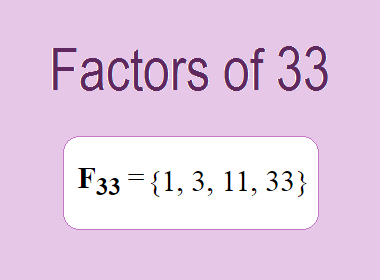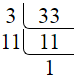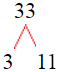# Factors of 33The factors of 33 are 1, 3, 11, and 33 i.e. F33 = {1, 3, 11, 33}. The factors of 33 are all the numbers that can divide 33 without leaving a remainder.

We can check if these numbers are factors of 33 by dividing 33 by each of them. If the result is a whole number, then the number is a factor of 33. Let's do this for each of the numbers listed above:

·        1 is a factor of 33 because 33 divided by 1 is 33.

·        3 is a factor of 33 because 33 divided by 3 is 11.

·        11 is a factor of 33 because 33 divided by 11 is 3.

·        33 is a factor of 33 because 33 divided by 33 is 1.

## How to Find Factors of 33?

1 and the number itself are the factors of every number. So, 1 and 33 are two factors of 33. To find the other factors of 33, we can start by dividing 33 by the numbers between 1 and 33. If we divide 33 by 2, we get a remainder of 1. Therefore, 2 is not a factor of 33. If we divide 33 by 3, we get a remainder of 0. Therefore, 3 is a factor of 33.

Next, we can check if 4 is a factor of 33. If we divide 33 by 4, we get a remainder of 1. Therefore, 4 is not a factor of 33. We can continue this process for all the possible factors of 33.

Through this process, we can find that the factors of 33 are 1, 3, 11, and 33. These are the only numbers that can divide 33 without leaving a remainder.

********************

********************

## Properties of the Factors of 33

The factors of 33 have some interesting properties. One of the properties is that the sum of the factors of 33 is equal to 48. We can see this by adding all the factors of 33 together:

1 + 3 + 11 + 33 = 48

Another property of the factors of 33 is that they are all odd numbers. This is because 33 is an odd number, and any even number cannot divide an odd number without leaving a remainder.

Another property of the factors of 33 is that the prime factors of 33 are 3 and 11 only.

## Applications of the Factors of 33

The factors of 33 have several applications in mathematics. One of the applications is in finding the highest common factor (HCF) of two or more numbers. The HCF is the largest factor that two or more numbers have in common. For example, to find the HCF of 33 and 22, we need to find the factors of both numbers and identify the largest factor they have in common. The factors of 33 are 1, 3, 11, and 33. The factors of 22 are 1, 2, 11, and 22. The largest factor that they have in common is 11. Therefore, the HCF of 33 and 22 is 11.

Another application of the factors of 33 is in prime factorization. Prime factorization is the process of expressing a number as the product of its prime factors. The prime factors of 33 are 3 and 11, since these are the only prime numbers that can divide 33 without leaving a remainder. Therefore, we can express 33 as:

33 = 3 × 11

We can do prime factorization by division and factor tree method also. Here is the prime factorization of 33 by division method,33 = 3 × 11

Here is the prime factorization of 33 by the factor tree method,33 = 3 × 11

## Conclusion

The factors of 33 are the numbers that can divide 33 without leaving a remainder. The factors of 33 are 1, 3, 11, and 33. The factors of 33 have some interesting properties, such as being odd numbers and having a sum of 48. The factors of 33 have several applications in mathematics, such as finding the highest common factor and prime factorization.